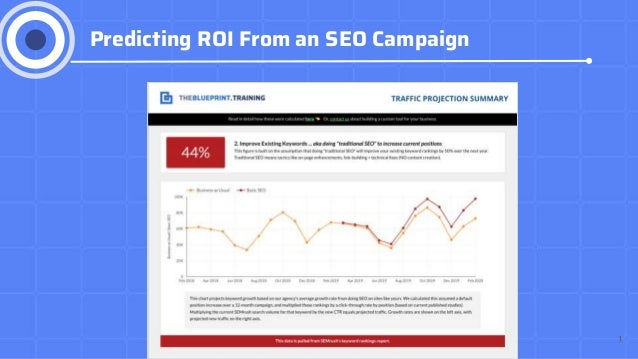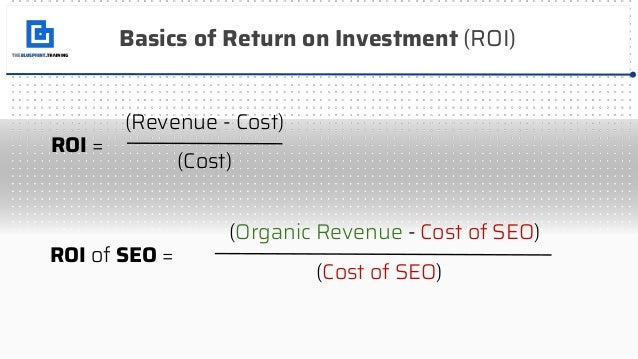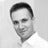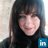Successfully reported this slideshow.Upcoming SlideShare
×

ofYouTube videos are no longer supported on SlideShareUpcoming SlideShare
Next

Share

# How to Calculate the ROI of SEO

This deck gives you detailed equations and data inputs to calculate the return on investment (ROI) of a Search Engine Optimization (SEO) campaign.

See all

See all

### How to Calculate the ROI of SEO

1. 1. 1 Predicting ROI From an SEO Campaign
2. 2. Basics of Return on Investment (ROI) ROI = (Revenue - Cost) (Cost) ROI of SEO = (Organic Revenue - Cost of SEO) (Cost of SEO)
3. 3. Finding ROI Inputs - COST Cost of SEO = ● The retainer fee / costs to an agency our vendor ● Cost of doing business in-house (freelancers, staff, etc) Cost of SEO = \$5,000/month or \$60,000/yearly
4. 4. Finding ROI Inputs - REVENUE Organic Revenue = ● Revenue generated from organic search marketing ● Can grab this from Google Analytics (or use lead data to calculate) Organic Revenue = Sales from Organic Search Channels
5. 5. We need to find PROJECTED ROI... ROI based on the amount of organic traffic we can drive, relative to the investment made in our services. ROI of SEO = (Projected Δ Organic Revenue - Cost of SEO) (Cost of SEO)
6. 6. We’ll do this for 2 types of sites... Ecommerce Lead Generation 1 2
7. 7. ROI (Projected Δ Organic Revenue - \$60,000) (\$60,000) Projected Δ Organic Revenue = ((Projected Organic Traffic x Conversion Rate) Projected Organic Traffic = (Current Organic Traffic x Growth Rate) (Current Organic Traffic)+ X (Average Order Value)) - Current Organic Revenue = 1
8. 8. Projected Organic Traffic = (Current Organic Traffic x Growth Rate) (Current Organic Traffic)+ 1
9. 9. 1 Behavior > Site Content > Landing Pages
10. 10. 1
11. 11. Projected Organic Traffic = (Current Organic Traffic x Growth Rate) (Current Organic Traffic)+ Projected Organic Traffic = (1,408,436 x .44) + (1,408,436) Projected Organic Traffic = 2,028,148
12. 12. Projected Δ Organic Revenue = ((Projected Organic Traffic x Conversion Rate) 2,028,148 X (Average Order Value)) - Current Organic Revenue 1
13. 13. Projected Δ Organic Revenue = ((2,028,148 x Conversion Rate) X (AOV)) 2,028,148 - Current Organic Revenue 1
14. 14. 1 Behavior > Site Content > Landing Pages
15. 15. Projected Δ Organic Revenue = ((2,028,148 x 1.75%) X (\$78.98)) - Current Organic Revenue 1 Projected Δ Organic Revenue = (\$2,797,525.72) - (\$1,944,167) Projected Δ Organic Revenue = \$853,359.04
16. 16. ROI (Projected Δ Organic Revenue - \$60,000) (\$60,000) Projected Δ Organic Revenue = = 1 \$853,359.04
17. 17. ROI (\$853,359.04 - \$60,000) (\$60,000) = 1 ROI = 966.70%
18. 18. ROI (Projected Δ Organic Revenue - Cost of SEO) (Cost of SEO) Projected Δ Organic Revenue = ((Projected Organic Traffic x Conversion Rate) X (Lead Value)) x Avg Sale Value = 2
19. 19. Projected Organic Traffic = (Current Organic Traffic x Growth Rate) (Current Organic Traffic)+ 1 Lead Value (Average Sale Value x Conversion Rate)= Current Organic Revenue = ((Current Organic Traffic x Conversion Rate) x Leads to Customers %) x Avg Sale Value
20. 20. 1 Behavior > Site Content > Landing Pages
21. 21. 1
22. 22. Projected Organic Traffic = (Current Organic Traffic x Growth Rate) (Current Organic Traffic)+ Projected Organic Traffic = (160,265 x .44) + (160,265) Projected Organic Traffic = 230,782
23. 23. Projected Δ Organic Revenue = ((230,782 x .02%) X (Lead Value)) x Current Organic Revenue 2
24. 24. 2 Lead Value (Average Sale Value x Conversion Rate)= Lead Value (\$60,000 x .0002)= Lead Value = \$12
25. 25. Projected Δ Organic Revenue = ((230,782 x .02%) X (\$12)) x Current Organic Revenue 2
26. 26. 2 Current Organic Revenue = ((Current Organic Traffic x Conversion Rate) x Leads to Customers %) x Avg Sale Value Current Organic Revenue = ((160,265 x .02%) x 20 %) x \$60,000 Current Organic Revenue = \$384,636
27. 27. Projected Δ Organic Revenue = ((230,782 x .02%) X (\$12)) - \$384,636 2 Projected Organic Revenue = \$553,876 Projected Δ Organic Revenue = \$169,239.84
28. 28. ROI (\$169,239.84 - \$60,000) (\$60,000) = 1 ROI = 111.55% ROI (Projected Δ Organic Revenue - Cost of SEO) = (Cost of SEO)
•#### DaReK_tHe_CrOw

Aug. 10, 2021
•#### PaytonMaurer

Apr. 13, 2021

Total views

3,314

On Slideshare

0

From embeds

0

Number of embeds

3,057

17

Shares

0